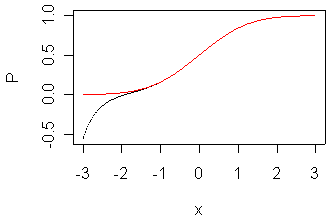# Computing normal probabilities

## 2005-05-19

One of the programs here at Children’s Mercy Hospital is helping me take an Excel spreadsheet and developing a Windows program that will do the same calculations faster and cleaner. The Excel spreadsheet uses the NORMSDIST function, which computes the probability that a standard normal distribution is less than or equal to a given value. Basically, it approximates the area under the bell shaped curve. He wanted to know what the formula was for this. It turns out there are a variety of formulas. StatLib has the Applied Statistics AS 66 algorithm, along with some competing algorithms at

Microsoft documents the NORMSDIST function on its web pages at

which references an Abramowitz and Stegun formula from the “Handbook of Mathematical Functions.” [BookFinder4U link]](http://www.bookfinder4u.com/detail/0486612724.html)

I took this algorithm and checked out the quality using R. Here’s the R code.

x <- seq(-3,3,0.01)
p <- 0.2316419
b1 <- 0.319381530
b2 <- -0.356563782
b3 <- 1.781477937
b4 <- -1.821255978
b5 <- 1.330274429
t <- 1/(1+p*x)
Z <- (1/(sqrt(2*pi))*exp(-1*x^2/2))
P <- 1 - Z * (b1*t+b2*t^2+b3*t^3+b4*t^4+b5*t^5)
par(mar=c(4.1,4.1,0.6,0.6))
plot(x,P,type="l")
lines(x,pnorm(x),col=2)
`

and here is the graph it produced.The red line represents the correct value and the black line is the Excel formula. Obviously this works well only for positive x values and maybe a few negative x values, but certainly not anything less than -1. There is a simple way to compute the probability for any negative value, of course, using the positive value.

You can find an earlier version of this page on my original website.